# Moment matrix

(diff) ← Older revision | Latest revision (diff) | Newer revision → (diff)

A matrix containing the moments of a probability distribution (cf. also Moment; Moments, method of (in probability theory)). For example, ifis a probability distribution on a set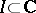, then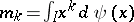is itsth order moment. Ifand thus the moments are given, then a linear functionalis defined on the set of polynomials by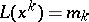,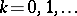. The inverse problem is called a moment problem (cf. also Moment problem): Given the sequence of moments,, find the necessary and sufficient conditions for the existence of and an expression for a positive distribution (a non-decreasing function with possibly infinitely many points of increase) that gives the integral representation of that linear functional. A positive distribution can only exist iffor any polynomialthat is positive on.

For the Hamburger moment problem (cf. also Complex moment problem, truncated),is the real axis and the polynomials are real, so the functionalis positive if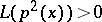for any non-zero polynomialand this implies that the moment matrices, i.e., the Hankel matrices of the moment sequence,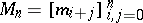, are positive definite for all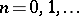(cf. also Hankel matrix). This is a necessary and sufficient condition for the existence of a solution.

For the trigonometric moment problem,is the unit circle in the complex plane and the polynomials are complex, so that "positive definite" here means that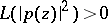for all non-zero polynomials. The linear functional is automatically defined on the space of Laurent polynomials (cf. also Laurent series) since. Positive definite now corresponds to the Toeplitz moment matrices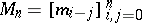being positive definite for all(cf. also Toeplitz matrix). Again this is the necessary and sufficient condition for the existence of a (unique) solution to the moment problem.

Once the positive-definite linear functional is given, one can define an inner product on the space of polynomials asin the real case or as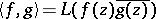in the complex case. The moment matrix is then the Gram matrix for the standard basis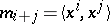or.

Generalized moments correspond to the use of non-standard basis functions for the polynomials or for possibly other spaces. Consider a set of basis functionsthat span the space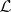. The modified or generalized moments are then given by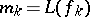. The moment problem is to find a positive distribution functionthat gives an integral representation of the linear functional on. However, to define an inner product, one needs the functional to be defined on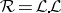(in the real case) or on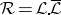(in the complex case). This requires a doubly indexed sequence of "moments"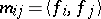. Finding a distribution for an integral representation ofon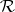is called a strong moment problem.

The solution of moment problems is often obtained using an orthogonal basis. If the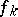are orthonormalized to give the functions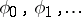, then the moment matrix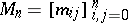can be used to give explicit expressions; namely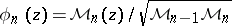where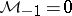,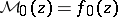and for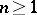,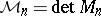with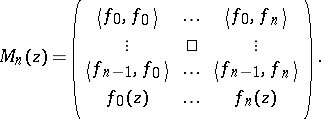The leading coefficient in the expansionsatisfies.